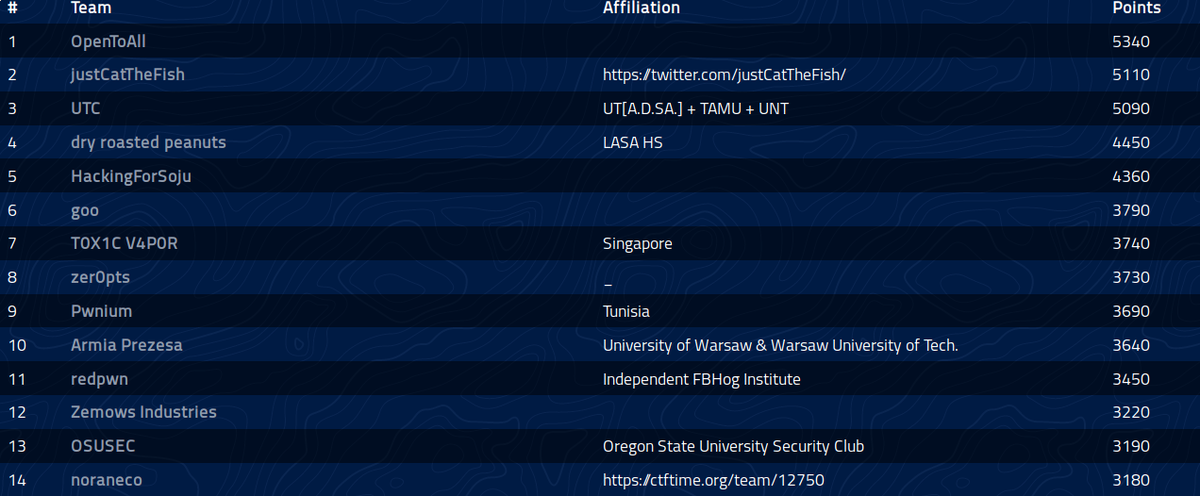# ångstromCTF 2019 writeup

I played ångstromCTF 2019 as a member of zer0pts. We gained 3730pts totally and got 8th place. I learned so many things from all the challenges. Thanks to all the admins!## [Rev 130pts] Icthyo

Long before stegosaurus roamed the earth, another species prowled the sea; here is an artist's rendition.

We were given two files: icthyo and out.png. ichtyo, which was a 64-bit ELF file, hid some texts into the image file. I decompiled it by ghidra and ovserbed the steganography process, then I found the interesting part of the code in encode function (I edited variable names to make it easier to understand the process) .

// (snipped)
printf("message (less than 256 bytes): ");
fgets((char *)msg,0x100,stdin);
y = 0;
while (y < 0x100) {
line = *(long *)(rows + (long)y * 8);
// (snipped)
i = 0;
while (i < 8) {
rgb = (byte *)(line + (long)(i * 0x60));
c = *(char *)((long)msg + (long)y);
if ((rgb & 1) != 0) {
rgb = rgb ^ 1;
}
rgb = rgb | (byte)((int)c >> ((byte)i & 0x1f)) & 1 ^ (rgb ^ *rgb) & 1;
i = i + 1;
}
y = y + 1;
}


In this process it sets the LSB to each bit of the character. So it could extract the hidden message by the following code. The hidden message was the flag: actf{lurking_in_the_depths_of_random_bits}.

from PIL import Image

img = Image.open("out.png")
w, h = img.size

lines = []

for y in range(h):
line = []
for x in range(w):
line.append(img.getpixel((x, y)))
lines.append(line)

buf = ""
for l in lines:
c = ""
for x in range(8):
r, g, b = l[x * 32]
c += str((b & 1) ^ ((r ^ g) & 1))
x = int(c[::-1], 2)
if x == 0:
break
buf += chr(x)
print(buf)


Note that the decompiled code processes the image byte by byte, whereas my code treated it for each pixels.

## [Crypto 100pts] Paint

This amazing new paint protocol lets artists share secret paintings with each other! Good thing U.S. Patent 4200770 is expired.

The values palette, base, my mix, your mix, and painting were given while secret and shared mix were hidden. They have the following relation.We need to get the shared mix to recover the image from the painting. Also, to get the shared mix We need to get the secret.

Then, how can I get the secret? Now, we know my mix, base and palette. So if the discrete log problemcould be solved, we will get the secret

Surprizingly, sage was able to do it.

my_mix = 68702...93113
base = 13489...86329
pallete = 32317...30656

secret = discrete_log(my_mix, Mod(base, pallete))
print(secret)

from Crypto.Util.number import *

secret = 62992...62361
pallete = 32317...30656
your_mix = 14317...48217
painting = 17665...43620

shared_mix = pow(your_mix, secret, pallete)
print(long_to_bytes(shared_mix ^ painting))


The flag was actf{powers_of_two_are_not_two_powerful}

## [Crypto 120pts]Secret Sheep Society

The sheep are up to no good. They have a web portal for their secret society, which we have the source for. It seems fairly easy to join the organization, but climbing up its ranks is a different story.

When we logged in to the web portal, the token was issued. Token consisted of an IV and a json encrypted with CBC mode AES. The json had two columns: admin and handle, and our mission is to make admin column true.

As the json was encrypted with CBC mode AES and the IV was given, we could tamper the first block of the plaintext by editing the iv.

import json
import base64

x = 'jirU9ZpEy+x0J9dOK1AOiU1rRsB+4cY9Hj8b19oQ/iYaK4zM0UdegzxK4dv7aYtv'
bs = bytearray(base64.b64decode(x))

bs[offset] = bs[offset] ^ ord('f') ^ ord(' ')
bs[offset+1] = bs[offset+1] ^ ord('a') ^ ord('t')
bs[offset+2] = bs[offset+2] ^ ord('l') ^ ord('r')
bs[offset+3] = bs[offset+3] ^ ord('s') ^ ord('u')
# bs[offset] = bs[offset] ^ ord('e') ^ ord('e')

print(base64.b64encode(bs))


## [Crypto 130pts]WALL-E

My friend and I have been encrypting our messages using RSA, but someone keeps intercepting and decrypting them! Maybe you can figure out what's happening?

from Crypto.Util.number import getPrime, bytes_to_long, inverse, long_to_bytes
# from secret import flag

flag = 'actf{' + '~'*(86-6) + '}'

assert(len(flag) < 87) # leave space for padding since padding is secure

p = getPrime(1024)
q = getPrime(1024)
n = p*q
e = 3
d = inverse(e,(p-1)*(q-1))
m = bytes_to_long(flag.center(255,"\x00")) # pad on both sides for extra security
c = pow(m,e,n)
print("n = {}".format(n))
print("e = {}".format(e))
print("c = {}".format(c))


Since e=3 and the flag was padded with "\x00", I tried the Coppersmith's attack.

from Crypto.Util.number import *
import string
n = 16930...08409
e = 3
c = 11517...26989

l = 86
pad_len = (255 - l) // 2

The flag was actf{bad_padding_makes_u_very_sadding_even_if_u_add_words_just_for_the_sake_of_adding}.